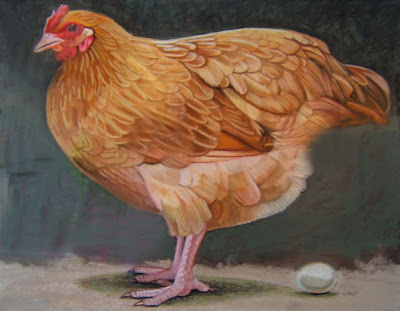## onsdag 5 oktober 2011

### The Hen and the Egg of Dark MatterThe 2011 Nobel Prize in Physics brings up the question of dark energy, which may not exist, while the other chamber of the Heart of Darkness, that is dark matter, may exist. This connects to the question of The Hen and Egg of Gravitation in Many-Minds Relativity presented as follows:

The gravitational potential P is related to distribution of matter M by the differential equation
• Laplace P = M
where Laplace is the Laplace operator. This equation can be read in two ways, with P the hen and M the matter:
1. Given M, the potential P is obtained by solving the differential equation Laplace P = M.
2. Given P, the matter distribution M is obtained by applying Laplace to P.
Here 1 is a non-local operation (solving a differential equation) reflecting action at distance, while 2 is a local operation (differentiation) without action at distance.

The usual approach is 1 with the complication of requiring spoky action at distance.

In the non-standard approach 2 there is no action at distance, and it also opens to an interpretation of matter as ordinary matter at points of singularity of Laplace P, where the potential P has "spikes", and dark matter where P is smooth (as Laplace P).

In this case the hen of the gravitational potential is the primary entity from which the egg of matter is generated. It may be easier to explain how a hen can lay an egg, than to describe how an egg may become a hen. In science the most efficient explanation is sought.

As concerns dark energy, it may be that inertia dominates on intergalatical scales and if so the present observed seemingly accelerating expansion may simply be a reflection of a Big Bang initial state with a certain expansion distribution without any need of any dark energy driving the expansion. Gravitation would then be important only on galactical scales. This fits with the observed lumpiness of the universe with large voids between galaxies.